# Basics of Javascript

27 de May de 2023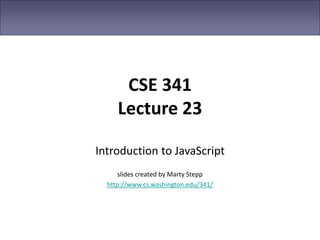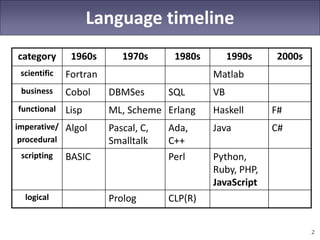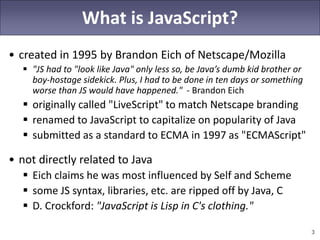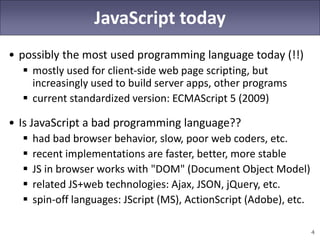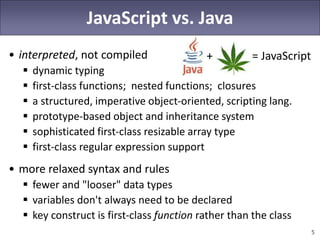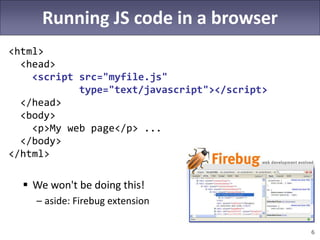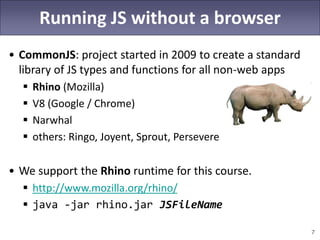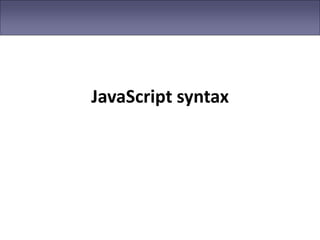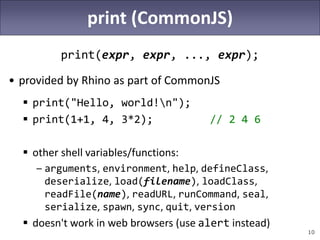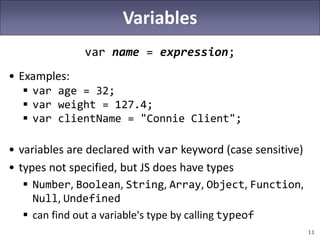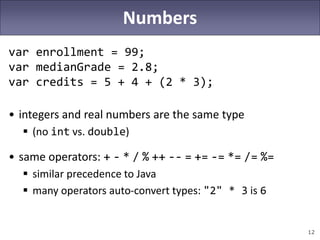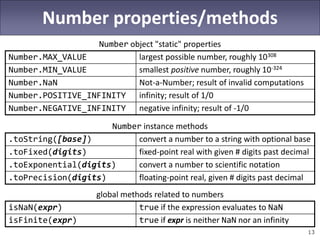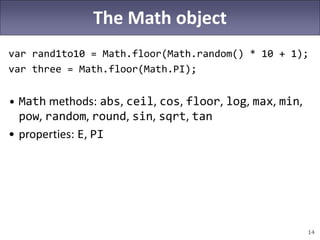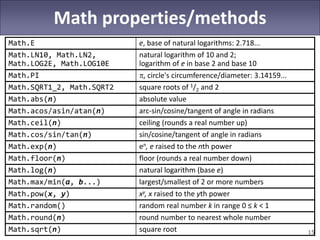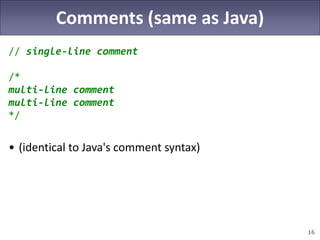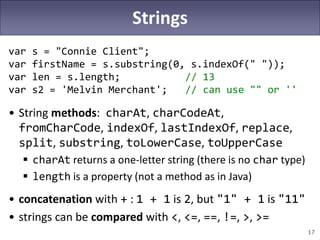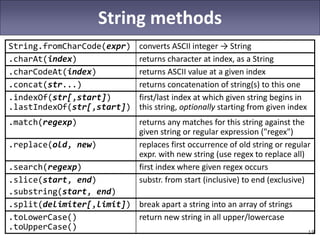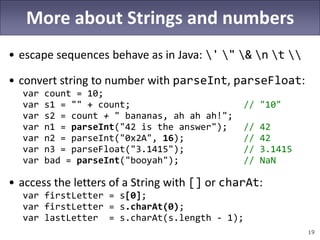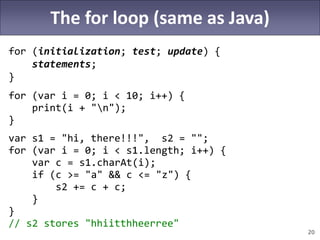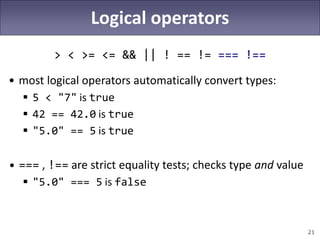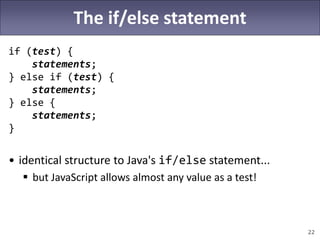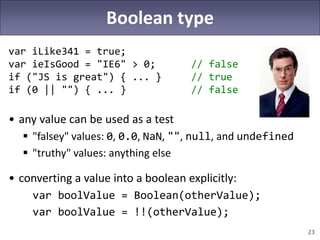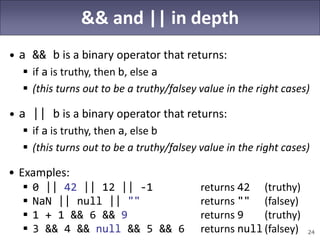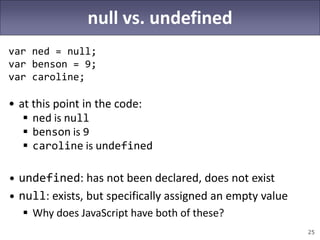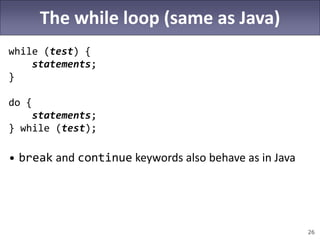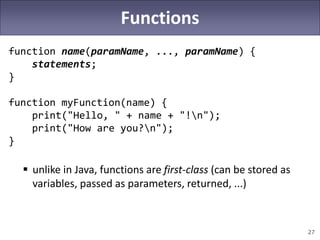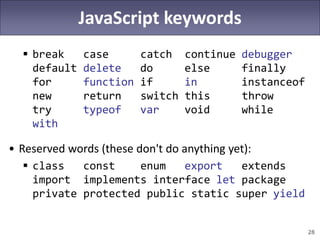1 de 28

### Basics of Javascript

• 1. CSE 341 Lecture 23 Introduction to JavaScript slides created by Marty Stepp http://www.cs.washington.edu/341/
• 2. 2 Language timeline category 1960s 1970s 1980s 1990s 2000s scientific Fortran Matlab business Cobol DBMSes SQL VB functional Lisp ML, Scheme Erlang Haskell F# imperative/ procedural Algol Pascal, C, Smalltalk Ada, C++ Java C# scripting BASIC Perl Python, Ruby, PHP, JavaScript logical Prolog CLP(R)
• 3. 3 What is JavaScript? • created in 1995 by Brandon Eich of Netscape/Mozilla  "JS had to "look like Java" only less so, be Java’s dumb kid brother or boy-hostage sidekick. Plus, I had to be done in ten days or something worse than JS would have happened." - Brandon Eich  originally called "LiveScript" to match Netscape branding  renamed to JavaScript to capitalize on popularity of Java  submitted as a standard to ECMA in 1997 as "ECMAScript" • not directly related to Java  Eich claims he was most influenced by Self and Scheme  some JS syntax, libraries, etc. are ripped off by Java, C  D. Crockford: "JavaScript is Lisp in C's clothing."
• 4. 4 JavaScript today • possibly the most used programming language today (!!)  mostly used for client-side web page scripting, but increasingly used to build server apps, other programs  current standardized version: ECMAScript 5 (2009) • Is JavaScript a bad programming language??  had bad browser behavior, slow, poor web coders, etc.  recent implementations are faster, better, more stable  JS in browser works with "DOM" (Document Object Model)  related JS+web technologies: Ajax, JSON, jQuery, etc.  spin-off languages: JScript (MS), ActionScript (Adobe), etc.
• 5. 5 JavaScript vs. Java • interpreted, not compiled  dynamic typing  first-class functions; nested functions; closures  a structured, imperative object-oriented, scripting lang.  prototype-based object and inheritance system  sophisticated first-class resizable array type  first-class regular expression support • more relaxed syntax and rules  fewer and "looser" data types  variables don't always need to be declared  key construct is first-class function rather than the class + = JavaScript
• 6. 6 Running JS code in a browser <html> <head> <script src="myfile.js" type="text/javascript"></script> </head> <body> <p>My web page</p> ... </body> </html>  We won't be doing this! – aside: Firebug extension
• 7. 7 Running JS without a browser • CommonJS: project started in 2009 to create a standard library of JS types and functions for all non-web apps  Rhino (Mozilla)  V8 (Google / Chrome)  Narwhal  others: Ringo, Joyent, Sprout, Persevere • We support the Rhino runtime for this course.  http://www.mozilla.org/rhino/  java -jar rhino.jar JSFileName
• 8. 8 The Rhino debugger java -classpath rhino.jar org.mozilla.javascript.tools.debugger.Main filename.js  http://www.mozilla.org/rhino/debugger.html
• 10. 10 print (CommonJS) print(expr, expr, ..., expr); • provided by Rhino as part of CommonJS  print("Hello, world!n");  print(1+1, 4, 3*2); // 2 4 6  other shell variables/functions: – arguments, environment, help, defineClass, deserialize, load(filename), loadClass, readFile(name), readURL, runCommand, seal, serialize, spawn, sync, quit, version  doesn't work in web browsers (use alert instead)
• 11. 11 Variables var name = expression; • Examples:  var age = 32;  var weight = 127.4;  var clientName = "Connie Client"; • variables are declared with var keyword (case sensitive) • types not specified, but JS does have types  Number, Boolean, String, Array, Object, Function, Null, Undefined  can find out a variable's type by calling typeof
• 12. 12 Numbers var enrollment = 99; var medianGrade = 2.8; var credits = 5 + 4 + (2 * 3); • integers and real numbers are the same type  (no int vs. double) • same operators: + - * / % ++ -- = += -= *= /= %=  similar precedence to Java  many operators auto-convert types: "2" * 3 is 6
• 13. 13 Number properties/methods Number object "static" properties Number.MAX_VALUE largest possible number, roughly 10308 Number.MIN_VALUE smallest positive number, roughly 10-324 Number.NaN Not-a-Number; result of invalid computations Number.POSITIVE_INFINITY infinity; result of 1/0 Number.NEGATIVE_INFINITY negative infinity; result of -1/0 Number instance methods .toString([base]) convert a number to a string with optional base .toFixed(digits) fixed-point real with given # digits past decimal .toExponential(digits) convert a number to scientific notation .toPrecision(digits) floating-point real, given # digits past decimal global methods related to numbers isNaN(expr) true if the expression evaluates to NaN isFinite(expr) true if expr is neither NaN nor an infinity
• 14. 14 The Math object var rand1to10 = Math.floor(Math.random() * 10 + 1); var three = Math.floor(Math.PI); • Math methods: abs, ceil, cos, floor, log, max, min, pow, random, round, sin, sqrt, tan • properties: E, PI
• 15. 15 Math properties/methods Math.E e, base of natural logarithms: 2.718... Math.LN10, Math.LN2, Math.LOG2E, Math.LOG10E natural logarithm of 10 and 2; logarithm of e in base 2 and base 10 Math.PI , circle's circumference/diameter: 3.14159... Math.SQRT1_2, Math.SQRT2 square roots of 1/2 and 2 Math.abs(n) absolute value Math.acos/asin/atan(n) arc-sin/cosine/tangent of angle in radians Math.ceil(n) ceiling (rounds a real number up) Math.cos/sin/tan(n) sin/cosine/tangent of angle in radians Math.exp(n) en, e raised to the nth power Math.floor(n) floor (rounds a real number down) Math.log(n) natural logarithm (base e) Math.max/min(a, b...) largest/smallest of 2 or more numbers Math.pow(x, y) xy, x raised to the yth power Math.random() random real number k in range 0 ≤ k < 1 Math.round(n) round number to nearest whole number Math.sqrt(n) square root
• 16. 16 Comments (same as Java) // single-line comment /* multi-line comment multi-line comment */ • (identical to Java's comment syntax)
• 17. 17 Strings var s = "Connie Client"; var firstName = s.substring(0, s.indexOf(" ")); var len = s.length; // 13 var s2 = 'Melvin Merchant'; // can use "" or '' • String methods: charAt, charCodeAt, fromCharCode, indexOf, lastIndexOf, replace, split, substring, toLowerCase, toUpperCase  charAt returns a one-letter string (there is no char type)  length is a property (not a method as in Java) • concatenation with + : 1 + 1 is 2, but "1" + 1 is "11" • strings can be compared with <, <=, ==, !=, >, >=
• 18. 18 String methods String.fromCharCode(expr) converts ASCII integer → String .charAt(index) returns character at index, as a String .charCodeAt(index) returns ASCII value at a given index .concat(str...) returns concatenation of string(s) to this one .indexOf(str[,start]) .lastIndexOf(str[,start]) first/last index at which given string begins in this string, optionally starting from given index .match(regexp) returns any matches for this string against the given string or regular expression ("regex") .replace(old, new) replaces first occurrence of old string or regular expr. with new string (use regex to replace all) .search(regexp) first index where given regex occurs .slice(start, end) .substring(start, end) substr. from start (inclusive) to end (exclusive) .split(delimiter[,limit]) break apart a string into an array of strings .toLowerCase() .toUpperCase() return new string in all upper/lowercase
• 19. 19 More about Strings and numbers • escape sequences behave as in Java: ' " & n t • convert string to number with parseInt, parseFloat: var count = 10; var s1 = "" + count; // "10" var s2 = count + " bananas, ah ah ah!"; var n1 = parseInt("42 is the answer"); // 42 var n2 = parseInt("0x2A", 16); // 42 var n3 = parseFloat("3.1415"); // 3.1415 var bad = parseInt("booyah"); // NaN • access the letters of a String with [] or charAt: var firstLetter = s; var firstLetter = s.charAt(0); var lastLetter = s.charAt(s.length - 1);
• 20. 20 The for loop (same as Java) for (initialization; test; update) { statements; } for (var i = 0; i < 10; i++) { print(i + "n"); } var s1 = "hi, there!!!", s2 = ""; for (var i = 0; i < s1.length; i++) { var c = s1.charAt(i); if (c >= "a" && c <= "z") { s2 += c + c; } } // s2 stores "hhiitthheerree"
• 21. 21 Logical operators > < >= <= && || ! == != === !== • most logical operators automatically convert types:  5 < "7" is true  42 == 42.0 is true  "5.0" == 5 is true • === , !== are strict equality tests; checks type and value  "5.0" === 5 is false
• 22. 22 The if/else statement if (test) { statements; } else if (test) { statements; } else { statements; } • identical structure to Java's if/else statement...  but JavaScript allows almost any value as a test!
• 23. 23 Boolean type var iLike341 = true; var ieIsGood = "IE6" > 0; // false if ("JS is great") { ... } // true if (0 || "") { ... } // false • any value can be used as a test  "falsey" values: 0, 0.0, NaN, "", null, and undefined  "truthy" values: anything else • converting a value into a boolean explicitly: var boolValue = Boolean(otherValue); var boolValue = !!(otherValue);
• 24. 24 && and || in depth • a && b is a binary operator that returns:  if a is truthy, then b, else a  (this turns out to be a truthy/falsey value in the right cases) • a || b is a binary operator that returns:  if a is truthy, then a, else b  (this turns out to be a truthy/falsey value in the right cases) • Examples:  0 || 42 || 12 || -1 returns 42 (truthy)  NaN || null || "" returns "" (falsey)  1 + 1 && 6 && 9 returns 9 (truthy)  3 && 4 && null && 5 && 6 returns null (falsey)
• 25. 25 null vs. undefined var ned = null; var benson = 9; var caroline; • at this point in the code:  ned is null  benson is 9  caroline is undefined • undefined: has not been declared, does not exist • null: exists, but specifically assigned an empty value  Why does JavaScript have both of these?
• 26. 26 The while loop (same as Java) while (test) { statements; } do { statements; } while (test); • break and continue keywords also behave as in Java
• 27. 27 Functions function name(paramName, ..., paramName) { statements; } function myFunction(name) { print("Hello, " + name + "!n"); print("How are you?n"); }  unlike in Java, functions are first-class (can be stored as variables, passed as parameters, returned, ...)
• 28. 28 JavaScript keywords  break case catch continue debugger default delete do else finally for function if in instanceof new return switch this throw try typeof var void while with • Reserved words (these don't do anything yet):  class const enum export extends import implements interface let package private protected public static super yield